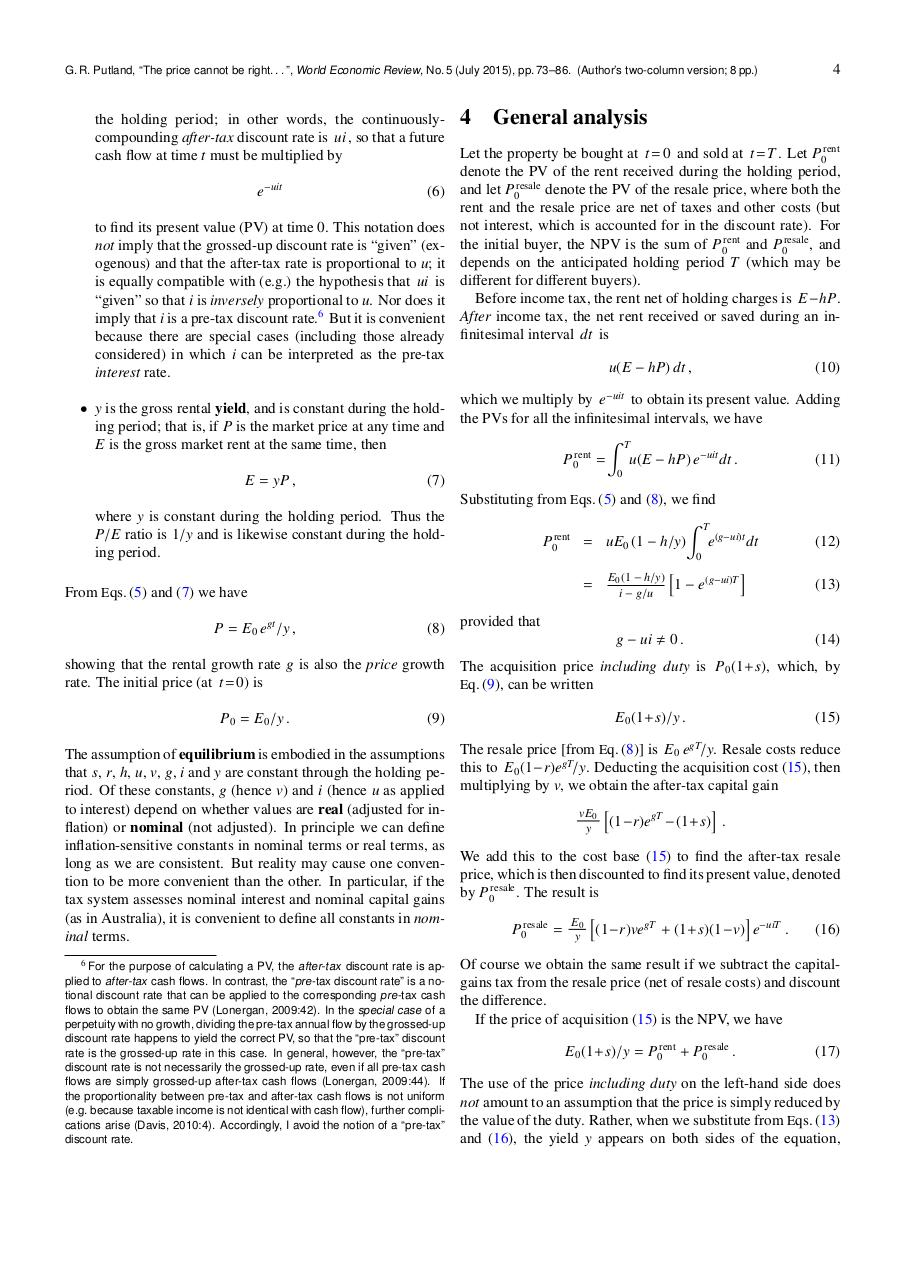PDF Archive

Easily share your PDF documents with your contacts, on the Web and Social Networks.

putland wer 5 2col.pdfPage 1 2 3 4 5 6 7 8

Text preview

G. R. Putland, “The price cannot be right. . . ”, World Economic Review, No. 5 (July 2015), pp. 73–86. (Author’s two-column version; 8 pp.)

4

the holding period; in other words, the continuously- 4 General analysis
compounding after-tax discount rate is ui , so that a future
Let the property be bought at t = 0 and sold at t = T . Let P0rent
cash flow at time t must be multiplied by
denote the PV of the rent received during the holding period,
e−uit
(6) and let P0resale denote the PV of the resale price, where both the
rent and the resale price are net of taxes and other costs (but
to find its present value (PV) at time 0. This notation does not interest, which is accounted for in the discount rate). For
not imply that the grossed-up discount rate is “given” (ex- the initial buyer, the NPV is the sum of P0rent and P0resale , and
ogenous) and that the after-tax rate is proportional to u; it depends on the anticipated holding period T (which may be
is equally compatible with (e.g.) the hypothesis that ui is different for different buyers).
Before income tax, the rent net of holding charges is E −hP.
“given” so that i is inversely proportional to u. Nor does it
6
After
income tax, the net rent received or saved during an inimply that i is a pre-tax discount rate. But it is convenient
finitesimal
interval dt is
because there are special cases (including those already
considered) in which i can be interpreted as the pre-tax
u(E − hP) dt ,
(10)
interest rate.
which we multiply by e−uit to obtain its present value. Adding
• y is the gross rental yield, and is constant during the holdthe PVs for all the infinitesimal intervals, we have
ing period; that is, if P is the market price at any time and
Z T
E is the gross market rent at the same time, then
rent
P0 =
u(E − hP) e−uit dt .
(11)
0
E = yP ,
(7)
Substituting from Eqs. (5) and (8), we find
where y is constant during the holding period. Thus the
Z T
P/E ratio is 1/y and is likewise constant during the holdP0rent = uE0 (1 − h/y) e(g−ui)t dt
(12)
ing period.
0
i
E (1 − h/y) h
1 − e(g−ui)T
(13)
= 0
From Eqs. (5) and (7) we have
i − g/u
P = E0 egt /y ,

(8)

showing that the rental growth rate g is also the price growth
rate. The initial price (at t = 0) is
P0 = E0 /y .

(9)

The assumption of equilibrium is embodied in the assumptions
that s, r, h, u, v, g, i and y are constant through the holding period. Of these constants, g (hence v) and i (hence u as applied
to interest) depend on whether values are real (adjusted for inflation) or nominal (not adjusted). In principle we can define
inflation-sensitive constants in nominal terms or real terms, as
long as we are consistent. But reality may cause one convention to be more convenient than the other. In particular, if the
tax system assesses nominal interest and nominal capital gains
(as in Australia), it is convenient to define all constants in nominal terms.
6 For the purpose of calculating a PV, the after-tax discount rate is applied to after-tax cash flows. In contrast, the “pre-tax discount rate” is a notional discount rate that can be applied to the corresponding pre-tax cash
flows to obtain the same PV (Lonergan, 2009:42). In the special case of a
perpetuity with no growth, dividing the pre-tax annual flow by the grossed-up
discount rate happens to yield the correct PV, so that the “pre-tax” discount
rate is the grossed-up rate in this case. In general, however, the “pre-tax”
discount rate is not necessarily the grossed-up rate, even if all pre-tax cash
flows are simply grossed-up after-tax cash flows (Lonergan, 2009:44). If
the proportionality between pre-tax and after-tax cash flows is not uniform
(e.g. because taxable income is not identical with cash flow), further complications arise (Davis, 2010:4). Accordingly, I avoid the notion of a “pre-tax”
discount rate.

provided that
g − ui , 0 .

(14)

The acquisition price including duty is P0 (1+ s), which, by
Eq. (9), can be written

E0 (1+ s)/y .

(15)

The resale price [from Eq. (8)] is E0 egT/y. Resale costs reduce
this to E0 (1−r)egT/y. Deducting the acquisition cost (15), then
multiplying by v, we obtain the after-tax capital gain
i
vE0 h
(1−r)egT −(1+ s) .
y
We add this to the cost base (15) to find the after-tax resale
price, which is then discounted to find its present value, denoted
by P0resale . The result is
i
E h
P0resale = y0 (1−r)vegT + (1+ s)(1−v) e−uiT .

(16)

Of course we obtain the same result if we subtract the capitalgains tax from the resale price (net of resale costs) and discount
the difference.
If the price of acquisition (15) is the NPV, we have
E0 (1+ s)/y = P0rent + P0resale .

(17)

The use of the price including duty on the left-hand side does
not amount to an assumption that the price is simply reduced by
the value of the duty. Rather, when we substitute from Eqs. (13)
and (16), the yield y appears on both sides of the equation,